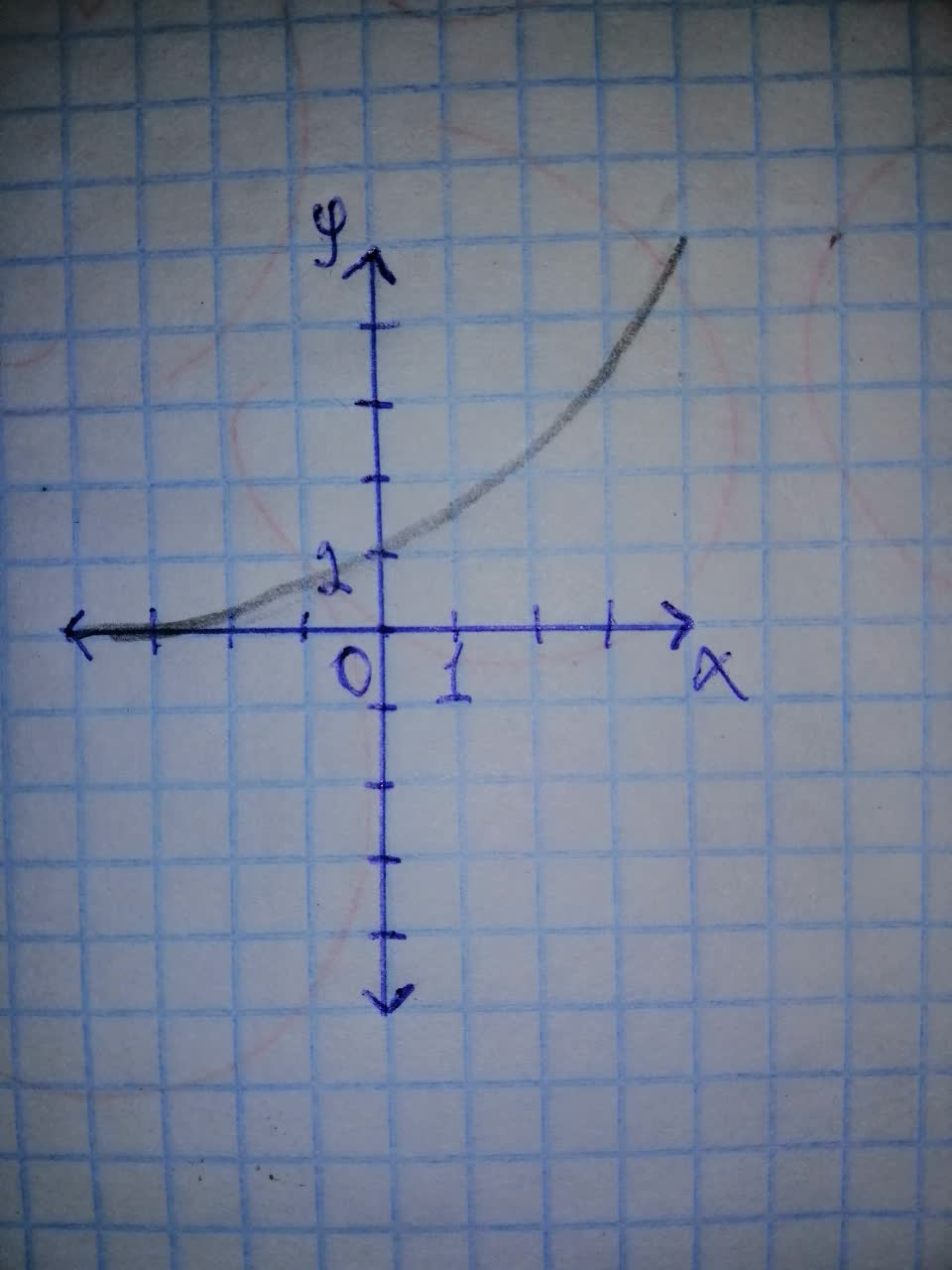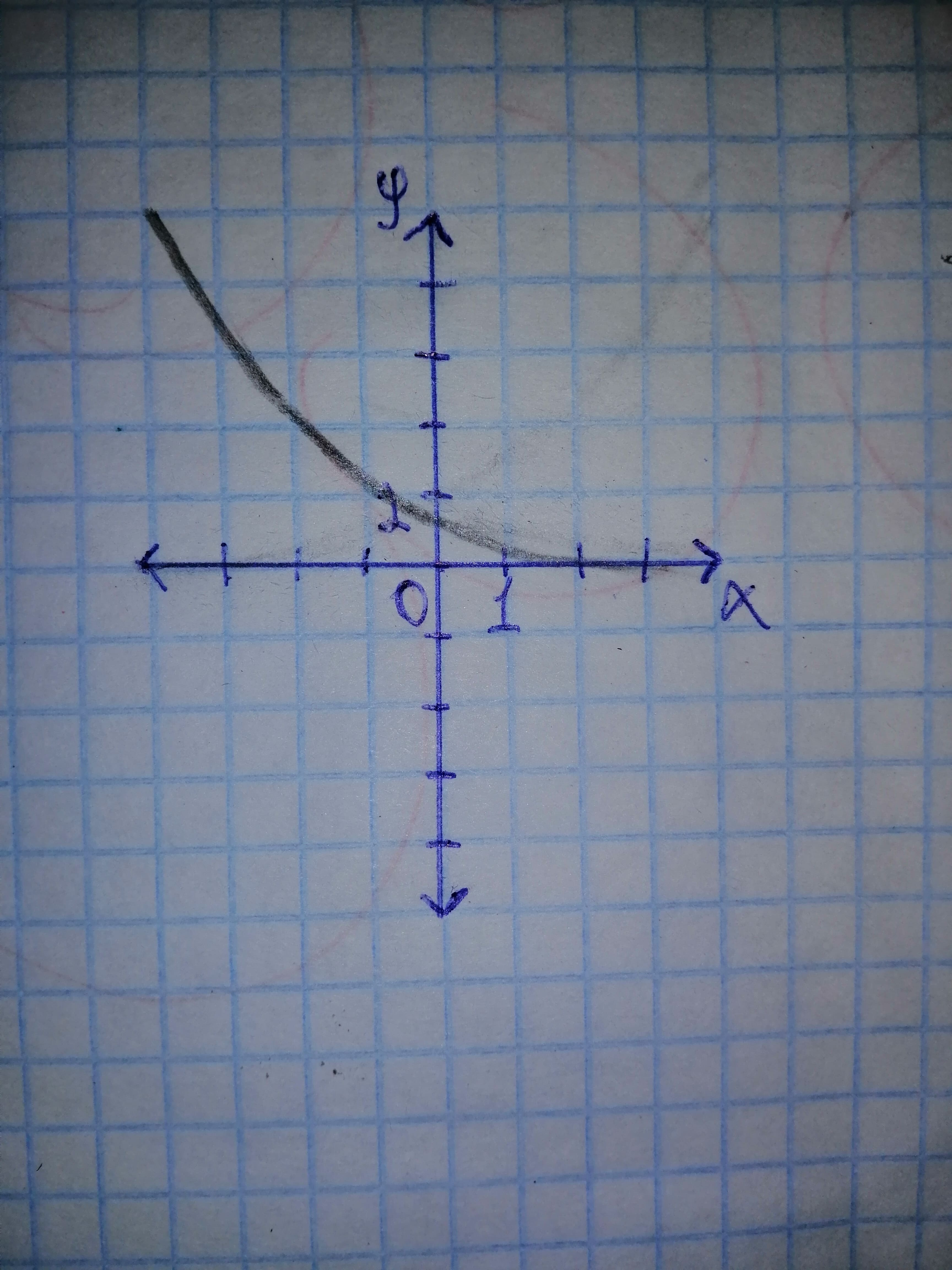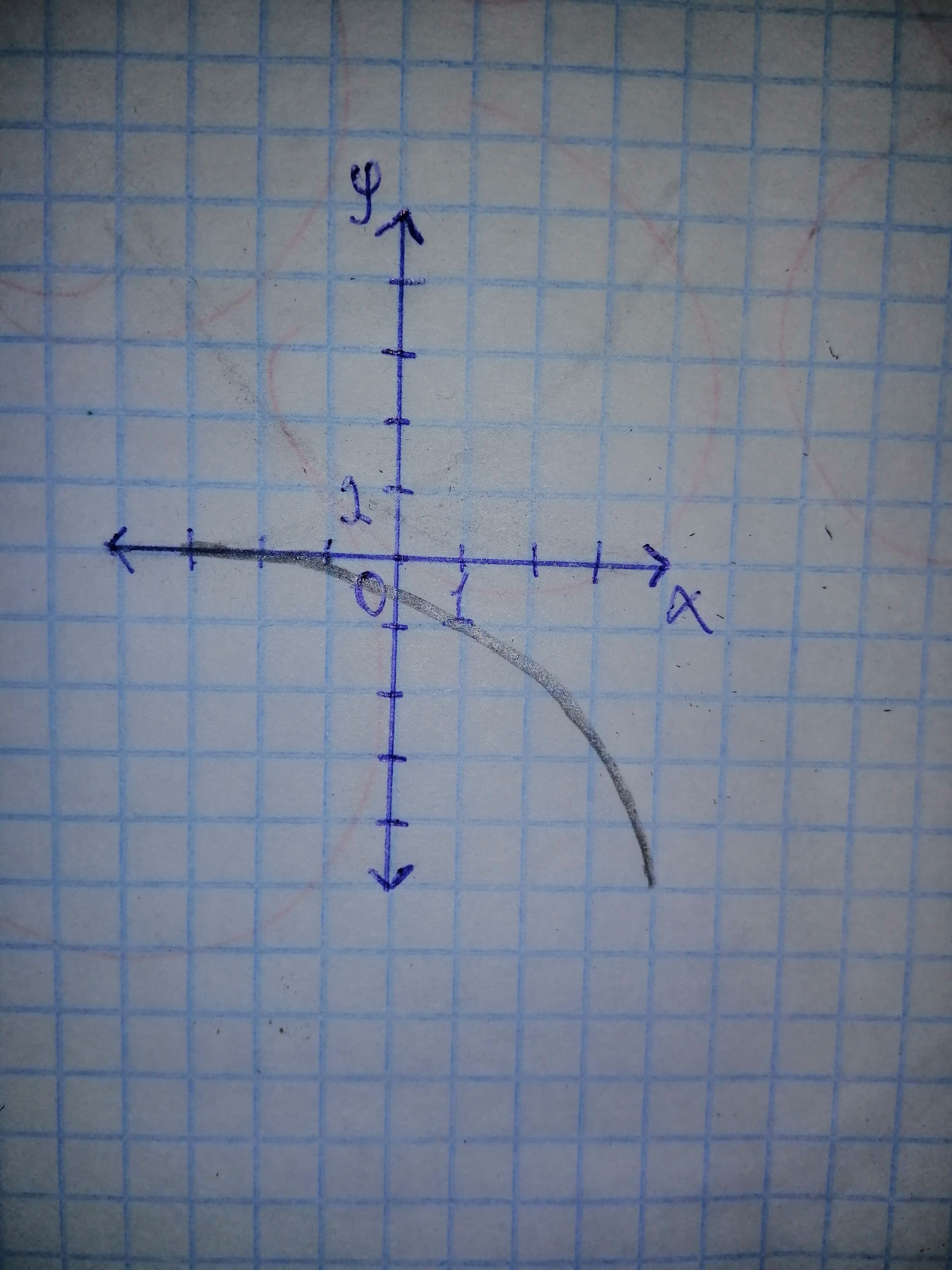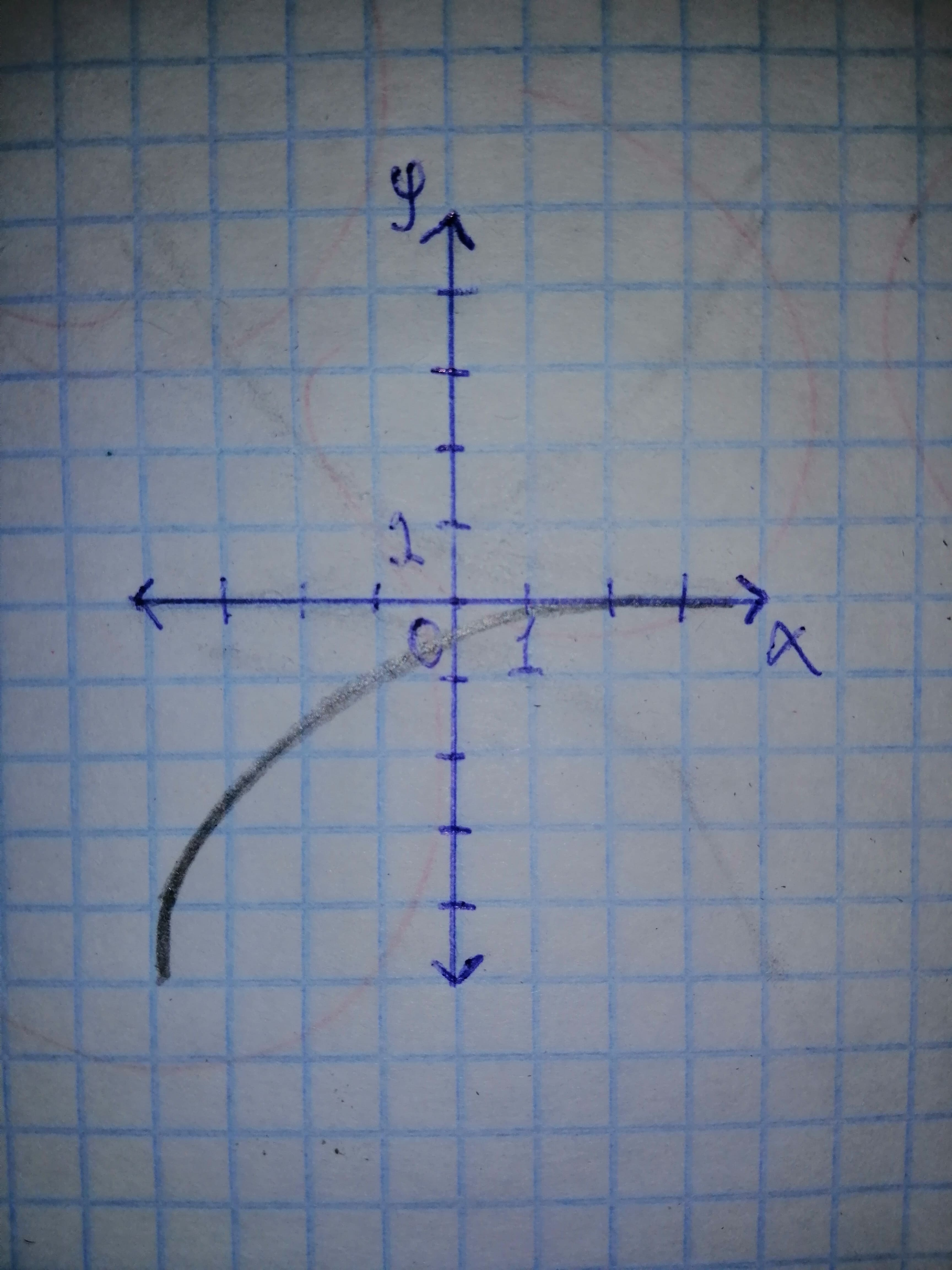# Draw a graph for each expression. a) f(x) = 2^{x} b) f(x) = 2^{-x} c) f(x) = - 2^{x} d) f(x) = -2^{-x}Dottie Parra 2021-01-06 Answered
Draw a graph for each expression.
a) $f\left(x\right)={2}^{x}$
b) $f\left(x\right)={2}^{-x}$
c)
d)
You can still ask an expert for help

• Questions are typically answered in as fast as 30 minutes

Solve your problem for the price of one coffee

• Math expert for every subject
• Pay only if we can solve itaveres8

Step 1
Properties of exponential function
1. Has continuous and smooth graph.
2. The graph passes through (0, 1) point.
3. The graph is increasing and it increases without bound.
4. Domain is whole real line.
5. Range is
Step 2
a) The graph of $f\left(x\right)={2}^{x}$ is:Step 3
b) The graph of $f\left(x\right)={2}^{-x}$ is obtained by taking reflection of the graph of
$f\left(x\right)={2}^{x}$ about y - axis.
Therefore, graph of $f\left(x\right)={2}^{-x}:$c) The graph of is obtained by taking reflection of the graph of
$f\left(x\right)={2}^{x}$ about x - axis.
Therefore, graph ofd) The graph of is obtained by taking reflection of the graph of
$f\left(x\right)={2}^{-x}$ about x - axis.
Therefore, graph of